# Excel IFERROR function with formula examples

The tutorial shows how to use IFERROR in Excel to catch errors and replace them with a blank cell, another value or a custom message. You will learn how to use the IFERROR function with Vlookup and Index Match, and how it compares to IF ISERROR and IFNA.

"Give me the place to stand, and I shall move the earth," Archimedes once said. "Give me a formula, and I shall make it return an error," an Excel user would say. In this tutorial, we won't be looking at how to return errors in Excel, we'd rather learn how to prevent them in order to keep your worksheets clean and your formulas transparent.

## Excel IFERROR function - syntax and basic uses

The IFERROR function in Excel is designed to trap and manage errors in formulas and calculations. More specifically, IFERROR checks a formula, and if it evaluates to an error, returns another value you specify; otherwise, returns the result of the formula.

The syntax of the Excel IFERROR function is as follows:

IFERROR(value, value_if_error)

Where:

• Value (required) - what to check for errors. It can be a formula, expression, value, or cell reference.
• Value_if_error (required) - what to return if an error is found. It can be an empty string (blank cell), text message, numeric value, another formula or calculation.

For example, when dividing two columns of numbers, you may get a bunch of different errors if one of the columns contains empty cells, zeros or text.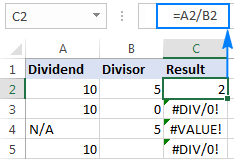To prevent that from happening, use the IFERROR function to catch and handle errors the way you want.

#### If error, then blank

Supply an empty string (") to the value_if_error argument to return a blank cell if an error is found:

`=IFERROR(A2/B2, "")`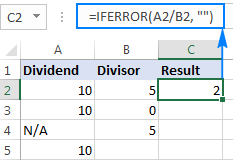#### If error, then show a message

You can also display your own message instead of Excel's standard error notation:

`=IFERROR(A2/B2, "Error in calculation")`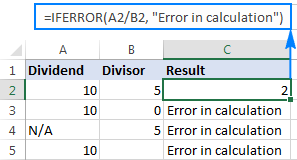### 5 things you should know about Excel IFERROR function

1. The IFERROR function in Excel handles all error types including #DIV/0!, #N/A, #NAME?, #NULL!, #NUM!, #REF!, and #VALUE!.
2. Depending on the contents of the value_if_error argument, IFERROR can replace errors with your custom text message, number, date or logical value, the result of another formula, or an empty string (blank cell).
3. If the value argument is a blank cell, it is treated as an empty string (''') but not an error.
4. IFERROR was introduced in Excel 2007 and is available in all subsequent versions of Excel 2010, Excel 2013, and Excel 2016.
5. To trap errors in Excel 2003 and earlier versions, use the ISERROR function in combination with IF, as shown in this example.

## IFERROR formula examples

The following examples show how to use IFERROR in Excel in combination with other functions to accomplish more complex tasks.

### Excel IFERROR with Vlookup

One of the most common uses of the IFERROR function is telling the users that the value they are searching for does not exist in the data set. For this, you wrap a VLOOKUP formula in IFERROR like this:

If the lookup value is not in the table you are looking in, a regular Vlookup formula would return the #N/A error: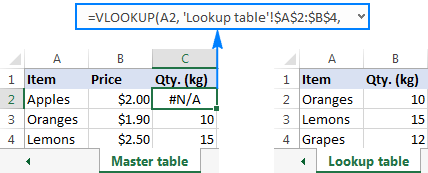For your users' piece of mind, wrap VLOOKUP in IFERROR and display a more informative and user-friendly message:

`=IFERROR(VLOOKUP(A2, 'Lookup table'!\$A\$2:\$B\$4, 2,FALSE), "Not found")`

The screenshot below shows this Iferror formula in Excel: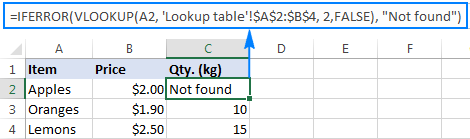If you'd like to trap only #N/A errors but not all errors, use the IFNA function instead of IFERROR.

For more Excel IFERROR VLOOKUP formula examples, please check out these tutorials:

### Nested IFERROR functions to do sequential Vlookups in Excel

In situations when you need to perform multiple Vlookups based on whether the previous Vlookup succeeded or failed, you can nest two or more IFERROR functions one into another.

Supposing you have a number of sales reports from regional branches of your company, and you want to get an amount for a certain order ID. With A2 as the lookup value in the current sheet, and A2:B5 as the lookup range in 3 lookup sheets (Report 1, Report 2 and Report 3), the formula goes as follows:

`=IFERROR(VLOOKUP(A2,'Report 1'!A2:B5,2,0),IFERROR(VLOOKUP(A2,'Report 2'!A2:B5,2,0),IFERROR(VLOOKUP(A2,'Report 3'!A2:B5,2,0),"not found")))`

The result will look something similar to this: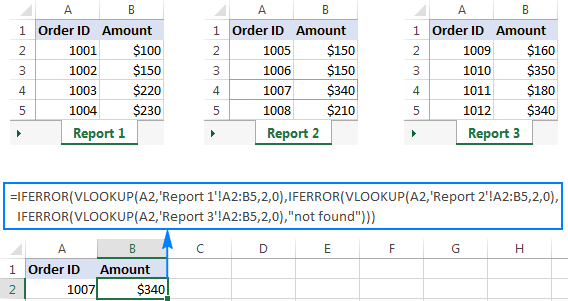For the detailed explanation of the formula's logic, please see How to do sequential Vlookups in Excel.

### IFERROR in array formulas

As you probably know, array formulas in Excel are meant to perform multiple calculations within a single formula. If you supply an array formula or expression that results in an array in the value argument of the IFERROR function, it'd return an array of values for each cell in the specified range. The below example shows the details.

Let's say, you have Total in column B and Price in column C, and you want to calculate Total Quantity. This can be done by using the following array formula, which divides each cell in the range B2:B4 by the corresponding cell of the range C2:C4, and then adds up the results:

`=SUM(\$B\$2:\$B\$4/\$C\$2:\$C\$4)`

The formula works fine as long as the divisor range does not have zeros or empty cells. If there is at least one 0 value or blank cell, the #DIV/0! error is returned: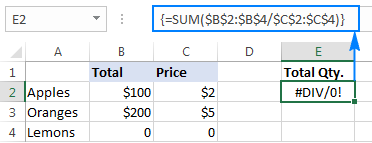To fix that error, simply do the division within the IFERROR function:

`=SUM(IFERROR(\$B\$2:\$B\$4/\$C\$2:\$C\$4,0))`

What the formula does is to divide a value in column B by a value in column C in each row (100/2, 200/5 and 0/0) and return the array of results {50; 40; #DIV/0!}. The IFERROR function catches all #DIV/0! errors and replaces them with zeros. And then, the SUM function adds up the values in the resulting array {50; 40; 0} and outputs the final result (50+40=90).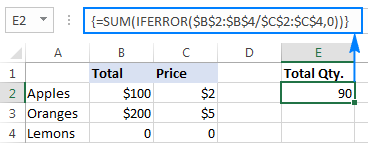Note. Please remember that array formulas should be completed by pressing the Ctrl + Shift + Enter shortcut.

## IFERROR vs. IF ISERROR

Now that you know how easy it is to use the IFERROR function in Excel, you may wonder why some people still lean towards using the IF ISERROR combination. Does it have any advantages compared to IFERROR? None. In the bad old days of Excel 2003 and lower when IFERROR did not exist, IF ISERROR was the only possible way to trap errors.  In Excel 2007 and later, it's just a bit more complex way to achieve the same result.

For instance, to catch Vlookup errors, you can use either of the below formulas.

In Excel 2007 - Excel 2016:

In all Excel versions:

Notice that in the IF ISERROR VLOOKUP formula, you have to Vlookup twice. In plain English, the formula can be read as follows: If Vlookup results in error, return "Not found", otherwise output the Vlookup result.

And here is a real-life example of an Excel If Iserror Vlookup formula:

`=IF(ISERROR(VLOOKUP(D2, A2:B5,2,FALSE)),"Not found", VLOOKUP(D2, A2:B5,2,FALSE ))`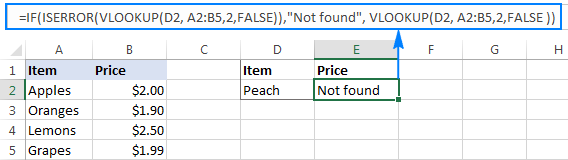## IFERROR vs. IFNA

Introduced with Excel 2013, IFNA is one more function to check a formula for errors.  Its syntax is similar to that of IFERROR:

IFNA(value, value_if_na)

In what way is IFNA different from IFERROR? The IFNA function catches only #N/A errors while IFERROR handles all error types.

In which situations you may want to use IFNA? When it is unwise to disguise all errors. For example, when working with important or sensitive data, you may want to be alerted about possible faults in your data set, and standard Excel error messages with the "#" symbol could be vivid visual indicators.

Let's see how you can make a formula that displays the "Not found" message instead of the N/A error, which appears when the lookup value is not present in the data set, but brings other Excel errors to your attention.

Supposing you want to pull Qty. from the lookup table to the summary table as shown in the screenshot below. Using the Excel Iferror Vlookup formula would produce an aesthetically pleasing result, which is technically incorrect because Lemons do exist in the lookup table: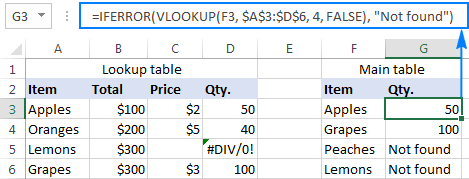To catch #N/A but display the #DIV/0 error, use the IFNA function in Excel 2013 and Excel 2016:

`=IFNA(VLOOKUP(F3,\$A\$3:\$D\$6,4,FALSE), "Not found")`

Or, the IF ISNA combination in Excel 2010 and earlier versions:

`=IF(ISNA(VLOOKUP(F3,\$A\$3:\$D\$6,4,FALSE)),"Not found", VLOOKUP(F3,\$A\$3:\$D\$6,4,FALSE))`

The syntax of the IFNA VLOOKUP and IF ISNA VLOOKUP formulas are similar to that of IFERROR VLOOKUP and IF ISERROR VLOOKUP discussed earlier.

As shown in the screenshot below, the Ifna Vlookup formula returns "Not found" only for the item that is not present in the lookup table (Peaches). For Lemons, it shows #DIV/0! indicating that our lookup table contains a divide by zero error: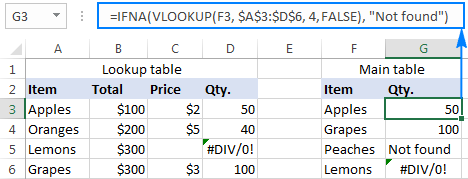## Best practices for using IFERROR in Excel

By now you already know that the IFERROR function is the easiest way to catch errors in Excel and mask them with blank cells, zero values, or custom messages of your own. However, that does not mean you should wrap each and every formula with error handling. The following simple recommendations may help you keep the balance.

1. Don't trap errors without a reason.
2. Wrap the smallest possible part of a formula in IFERROR.
3. To handle only specific errors, use an error handling function with a smaller scope:
• IFNA or IF ISNA to catch only #N/A errors.
• ISERR to catch all errors except for #N/A.

This is how you use the IFERROR function in Excel to trap and handle errors. To have a closer look at the formulas discussed in this tutorial, you are welcome to download our sample IFERROR Excel workbook. I thank you for reading and hope to see you on our blog next week.

## You may also be interested in

### 22 responses to "Excel IFERROR function with formula examples"

1.rohan says:

Thanks for informatiom

2.Ananad Kumar says:

Dear Sir/Team
I have make an excel sheet of my business ledger. But in this worksheet "Iferror & Index" formula not working after 499 rows, and nothing shown any result after 499 rows. Is this formula only worked upto 499 rows or not? Can I increase the rows above 499? If I can increase the row after 499, then what should I do? Please tell me.
Thanks

•Gennady Terekhov (Ablebits.com Team) says:

Hello,
For me to understand the problem better, please send me a small sample workbook with your source data and the result you expect to get to support@ablebits.com. Please don't worry if you have confidential information there, we never disclose the data we get from our customers and delete it as soon as the problem is resolved.

3.Renny says:

Please Help. I need to track # of homes by Month (Column), by Community # (Row), from my boss's worksheet (2018 starts.xlsx) to my own. It pulls in the right information, but every time I open my spreadsheet, my computer can't seem to function properly. an Intern helped with the formula, and she's unreachable.

Any help is greatly appreciated. Thank you!

=IFERROR(IF(LEN(INDEX('[2018 starts.xlsx]Labor North'!\$1:\$1048576,MATCH(\$B7,'[2018 starts.xlsx]Labor North'!\$C:\$C,0),MATCH(P\$5,'[2018 starts.xlsx]Labor North'!\$2:\$2,0))),INDEX('[2018 starts.xlsx]Labor North'!\$1:\$1048576,MATCH(\$B7,'[2018 starts.xlsx]Labor North'!\$C:\$C,0),MATCH(P\$5,'[2018 starts.xlsx]Labor North'!\$2:\$2,0)),""),"")

4.Manny says:

Hello. I have a file with data in 4 columns. Some of the data may be repeated between columns. How can I create a list in column 5 with unique numbers from the other 4?

Any help is greatly appreciated. Thank you!

5.Patrick says:

Great synopsys. Thanks

6.Shilpa says:

Nice information it helps all computer science students

7.Dilip Dighe says:

Dear Sir/Mam
very helpful & thankful yours guideline & thank u so much....

I Create one table... I M nt sure which is formula this table...
plz. help me..

Table (FOR EXAMPLE)

1 A B C D E F
2 Sr.No Name Item Qty. Rate Total
3 1 Abc Box 50 100 5000
4 2 Abc Box 20 50 1000
5 3 Cbd Box 20 50 1000
6 4 Dcd Box 20 50 1000

I Enter (Lookup Value)Other Sheet - Sr.No.1 &
Result is :- (TABLE)Below

Enter No. 1
Name = Abc (Vlookup Value)
1 A B C D
2 Item Qty. Rate Total
3 Box 50 100 5000
4 Box 20 50 1000

plz. help me..

Thnking you
Dilip

8.Nabeel says:

9.Shravan says:

Hello Sir/Mam,

i am create a scorecard for my employ's. i have fixed target and tracking daily basis achievement.
for example.

Target = 6, (E2) Column, Achievement = 0, (F2) Column. i am used if formula =IFERROR (IF((20*(E2/F2)>20),20,(20*(E2/F2))),"20.0")

value showing is (20.0) but i am use sum function Parameter-1 value is (20.0) and parameter-2 value is (15.0) both value sum = (35.0) but showing is (15.0)

10.Josephine says:

What is the formula of; when u create another excel for overall charts and u need to sum or average it, and make sure that theres no result yet will not appear on the chart u create.

11.Pet says:

Hello everyone - just need some help please
COLUMN A = Response Sent Date
COLUMN B = Last Update Date
OUTPUT - I need to calculate how many working days from COLUMN B; however if COLUMN B is Blank or no date, it will refer to COLUMN A's date

12.Ishtiaq Ali Syed says:

Please I have to know about ifferror() ,how to apply in excel 2009,?

13.Babu says:

Dear All,
Kindly help me for this below table looking for formula. Next sheet if i click the any names it should get the data...
Sl. No. NAMES DAY DATE Business Purchase Amount
1 A Monday 2-Sep-19 1000
2 B Tuesday 4-Sep-19 1050
3 C Wednesday6-Sep-19 1500
4 D Thursday8-Sep-19 950
5 E Friday 10-Sep-19 1360
6 A Saturday12-Sep-19 490
7 B Sunday 14-Sep-19 1485
8 C Monday 16-Sep-19 620
9 D Tuesday 18-Sep-19 9563
10 E Wednesday20-Sep-19 623

14.saravana says:

Nice Information to grow the Knowledge.

15.Munawar says:

A1 1
A2 2
A3 ERROR
A4 4
if we do sum(A1:A4), sum should'nt work, its there any way

16.David says:

I have a formula that is working, but it is not giving me a true picture.
This is the current cell(B5) formula: =IFERROR(IF(AND(AA5="",AJ5=""),"",\$A\$2-\$AJ5),"")
I need this cell(B5) to be BLANK if the adjacent cell(C5) is populated. C5 has a formula in it that gives me a number or a BLANK.

•Alexander Trifuntov (Ablebits.com Team) says:

Hello David!
If I understand your task correctly, the following formula should work for you:

=IF(C5<>"",IFERROR(IF(AND(AA5="",AJ5=""),"",\$A\$2-\$AJ5),""),"")

I hope this will help

17.Jagjit Rai says:

1 HC Max 36 2 Current HC 3 Performance-(THese are 3 headings)
17 15 2 Over HC Correct answer
Not played N/A N/A Over HC In correct- only need (N/A) Remove Over HC
36 36 0FALSE Incorrect- only need (0) Remove FALSE
Deae Sirs,
I have 3 columns as shown above ,and am subtracting column 2 from column 1 to get the following answers in column 3:-
1)Result to show "Nr Over HC" as 1 or 2 or 3 with remaks as " nr Over HC"-this is correct
2)Result to show N/A only But I get "N/A Over HC" together-This is not Correct.
3)Result to show 0 only But I get 0FALSE- This is not correct
I will be grateful if yu can help please.
Regards,
Jagjit

18.Jagjit Rai says:

=IFERROR(BK4-BM4,"N/A")&IF(BK4>BM4," Over HC")
Sorry I forgot to show you my Formula.
Regards,
Jagjit

19.Stephen Ochieng says:

Albeits.com is dong a great job
bravo!!